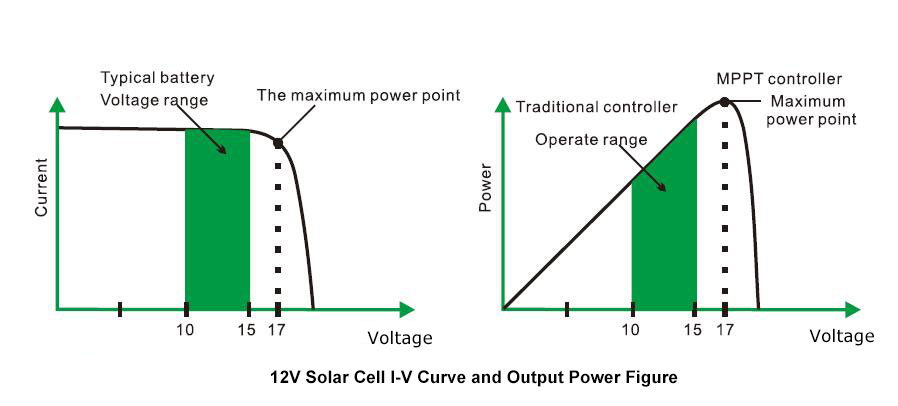# Why You Need a MPPT Solar Charge Controller?

Why do you need a MPPT solar charge controller for your PV system? For its excellent technology features, and for its huge advantages over other kinds of charge controller, MPPT charge controller is your first choice right now.

Features of Maximum Power Point Tracking

In most cases, the maximum power point tracking technology will increase the charging current of the system. Assuming that a system may have 10 amps of current flowing from the solar array into the MPPT charge controller, then there will be 12 amps current flowing from the MPPT controller to the battery. MPPT charge controller does not generate current. The input energy to the MPPT controller is equal to its input energy. Since the power (Watt) is the production of voltage and current (Volt x Ampere), the following equation would be established:

1. MPPT charge controller input power = MPPT charge controller output power
2. Input voltage x input current = output voltage x output current
* Suppose that the efficiency is 100%, ignoring the power loss of during wire and conversion.

If the voltage Vmp of the maximum power point of the solar array is larger than the battery voltage, the battery charge current must be proportionally larger than the output current of solar array, so that the input and output power can be balanced. The greater difference between the Vmp voltage and the battery voltage, the greater current enhancement. Current enhancement is extremely important in the PV system, because the maximum power point Vmp voltage of solar panel in a PV system is usually higher than the battery voltage.

Advantages of MPPT solar charge controller

The traditional charge controllers have the solar array be directly connected to the battery when charging, and it requires the solar array usually operates below the Vmp voltage range. Take a 12V system as example, the battery voltage range usually is 11- 15V, and the Vmp voltage of the solar array is usually about 16V or 17V. See the below figure, showing the current, voltage and output power curves of the nominal voltage 12V off grid solar cell.The maximum power point voltage of solar PV array is the maximum voltage of out power (Ampere x Voltage), and it is in the highest point of the I-V curve as shown in the left figure above. The traditional controller does not always operate at the Vmp of solar array, so the energy is wasted which could have been used to charge the battery and supply power to the system load. The greater difference between the battery voltage and the Vmp of the solar array, the more energy can be wasted. The MPPT solar charge controller will always run with the maximum power point, which reduce the waste of energy compared with the traditional controller.

Factors that affect the efficiency of MPPT solar charge controllerThe voltage Vmp of the solar PV array will decrease as the temperature of the array increases. In hot weather, Vmp may be close to or even lower than the battery voltage. In this case, the MPPT controller will obtain a few or even no energy compared to traditional controller. However, as long as the nominal voltage of the PV module is higher than the battery voltage, the Vmp of the PV module will always be higher than the battery voltage. In addition, the MPPT solar charge controller has a significant advantage even in hot weather, since it reduces the current of solar array and saves the wiring.

Our ATO-MPPT series of solar charge controller utilizes MPPT (maximum power point tracking) technology to extract the maximum power from the solar array to charge the batteries. The maximum power point tracking mode is full automatic and the user doesn't need adjustment. When the maximum power point of the array changes with ambient conditions, the charge controller automatically tracks the maximum power point of the array to ensure that the maximum energy of a day is obtained from the solar array. The MPPT solar charge controllers range from 20 Amp to 60 Amp with great advantages.

• Excellent heat dissipation design and intelligent cooling fan.
• Advanced maximum power tracking technology can significantly improve the utilization rate of PV system, the conversion rate up to 97%.
• Fast scan the entire I-V curve to track the maximum power point in a few second.
• Sealed, colloid, open lead-acid battery and lithium battery charging program is optional.
• Has automatic protection function for overcharge, over discharge, overload, short circuit, etc.